# Samacheer Kalvi 10th Maths Solutions Chapter 8 Statistics and Probability Ex 8.5

## Tamilnadu Samacheer Kalvi 10th Maths Solutions Chapter 8 Statistics and Probability Ex 8.5

Multiple Choice Questions
Question 1.
Which of the following is not a measure of dispersion?
(1) Range
(2) Standard deviation
(3) Arithmetic mean
(4) Variance
Solution:
(3) Arithmetic meanQuestion 2.
The range of the data 8, 8, 8, 8, 8. . . 8 is _____
(1) 0
(2) 1
(3) 8
(4) 3
(1) 0
Hint:
Range = L – S = 8 – 8 = 0

Question 3.
The sum of all deviations of the data from its mean is
(1) Always positive
(2) always negative
(3) zero
(4) non-zero integer
Solution:
(3) zero

Question 4.
The mean of 100 observations is 40 and their standard deviation is 3. The sum of squares of all deviations is ______
(1) 40000
(2) 160900
(3) 160000
(4) 30000
(2) 160900
Hint: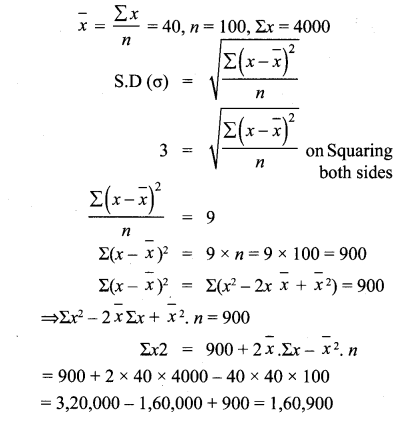Question 5.
Variance of the first 20 natural numbers is
(1) 32.25
(2) 44.25
(3) 33.25
(4) 30
Solution:
(3) 33.25

Question 6.
The standard deviation of a data is 3. If each value is multiplied by 5 then the new variance is ______
(1) 3
(2) 15
(3) 5
(4) 225
(4) 225
Hint:
Standard deviation = 3
Each value is multiplied by 5
New standard deviation = 3 × 5 = 15
New variance = 152 = 225

Question 7.
If the standard deviation of x, y, z is p then the standard deviation of 3x + 5, 3y + 5, 3z + 5 is
(1) 3p + 5
(2) 3p
(3) p + 5
(4) 9p + 15
Solution:
(2) 3pQuestion 8.
If the mean and coefficient of variation of a data are 4 and 87.5% then the standard deviation is _____
(1) 3.5
(2) 3
(3) 4.5
(4) 2.5
(1) 3.5
Hint: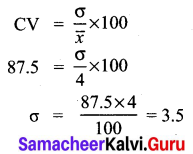Question 9.
Which of the following is incorrect?
(1) P (A) > 1
(2) 0 ≤ P(A) ≤ 1
(3) P(ϕ) = 0 (4)
(4) P (A) + P($$\overline{\mathbf{A}}$$) = 1
Solution:
(1) P(A) > 1

Question 10.
The probability a red marble selected at random from a jar containing p red, q blue and r green marbles is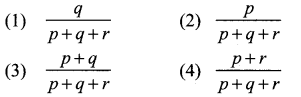Solution:
(2) $$\frac{p}{p+q+r}$$Question 11.
A page is selected at random from a book. The probability that the digit at units place of the page number chosen is less than 7 is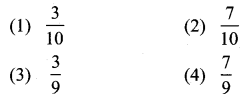Solution:
(2) $$\frac{7}{10}$$

Question 12.
The probability of getting a job for a person is $$\frac{x}{3}$$. If the probability of not getting the job is $$\frac{2}{3}$$ then the value of x is ____
(1) 2
(2) 1
(3) 3
(4) 1.5
(2) 1
Hint: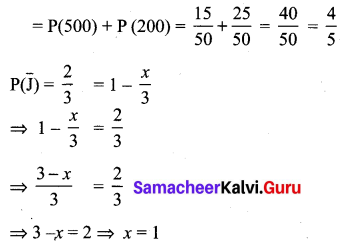Question 13.
Kamalam went to play a lucky draw contest. 135 tickets of the lucky draw were sold. If the probability of Kamalam winning is $$\frac{1}{9}$$, then the number of tickets bought by Kamalam is
(1) 5
(2) 10
(3) 15
(4) 20
Solution:
(3) 15
Hint:
$$=\frac{1}{9} \times 135=15$$Question 14.
If a letter is chosen at random from the English alphabets {a, b, ……, z} then the probability that the letter chosen precedes x _______
(1) $$\frac{12}{13}$$
(2) $$\frac{1}{13}$$
(3) $$\frac{23}{26}$$
(4) $$\frac{3}{26}$$
(3) $$\frac{23}{26}$$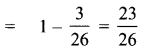(1) $$\frac{1}{5}$$
(2) $$\frac{3}{10}$$
(3) $$\frac{2}{3}$$
(4) $$\frac{4}{5}$$
(4) $$\frac{4}{5}$$